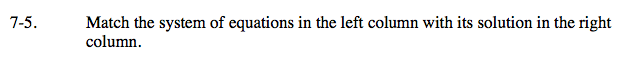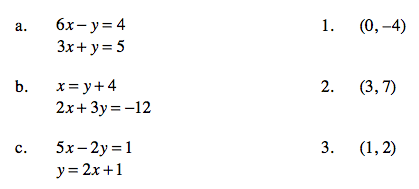Home > CAAC > Chapter 7 > Lesson 7.1.1 > Problem7-5

7-5.
1. Match the system of equations with its solution. Homework Help ✎

2.  a. 6x − y = 4 3x + y = 5 1. (0, –4) b. x = y + 4 2x + 3y = −12 2. (3, 7) c. 5x − 2y = 1 y = 2x + 1 3. (1, 2)One way is to substitute the points in the right column into the equations in the left column and find which point (1, 2, or 3) works with which system of equations (a, b, or c).

Another way is to solve the system and look for the solution.

(a) matches to (3).